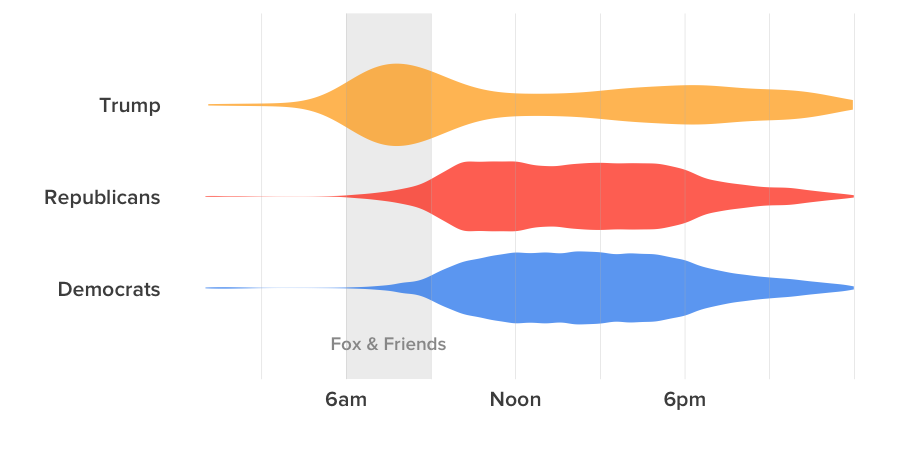# All The President’s Tweets — And Every Lawmaker’s Too

Data and R code for the analysis supporting this Jan. 23, 2018 BuzzFeed News post on political Twitter in the first year of Donald Trump’s presidency. Supporting files are in this GitHub repository.

### Data

We gathered data on tweets from Donald Trump’s personal Twitter account, and for the official accounts of all members of Congress, using the Twitter API. We identified congressional accounts from data maintained by theunitedstates.io, which includes Twitter handles, and joined this data to the tweets data to identify members by party. Given the limit of 3,200 tweets when pulling from users’ timelines, we harvested data multiple times over the course of the year to obtain data from the most active accounts.

On Jan. 20, 2018 at 3 p.m. Eastern time, we also gathered basic information for each account from the Twitter API, including follower counts.

### Setting up

Required packages, color palettes, and regular expressions to use for parsing tweets.

``````# load required packages
library(tidytext)
library(dplyr)
library(tidyr)
library(ggplot2)
library(lubridate)
library(stringr)
library(wordcloud)
library(DT)

# palette for Congressional Democrats and Republicans, plus Trump
party_pal <- c("#1482EE","#FF3300", "#FFA500")

# palettes for wordclouds
dem_pal <- c("#47B5FF", "#1482EE", "#004FBB")
rep_pal <-  c("#FF6633", "#FF3300", "#CC0000")
trump_pal <-  c("#FFD833", "#FFA500", "#CC7200")

# regexes for parsing tweets using the tidytext package
replace_reg <- "https?://[^\\s]+|&amp;|&lt;|&gt;|\\bRT\\b"
unnest_reg <- "([^A-Za-z_\\d#@']|'(?![A-Za-z_\\d#@]))"``````

The two Independent Senators, Bernie Sanders of Vermont and Angus King of Maine, caucus with the Democrats. So we assigned them as Democrats for this analysis, then created new variables for `time` (US Eastern) and `category`, to group by party and Trump.

``````tweets <- data_frame()

files <- list.files("data/tweets")
for (f in files) {
tmp <- read_csv(paste0("data/tweets/",f), col_types = cols(
.default = col_character(),
favorited = col_logical(),
favoriteCount = col_integer(),
retweetCount = col_integer(),
created = col_datetime(format = ""),
truncated = col_logical(),
isRetweet = col_logical(),
retweeted = col_logical(),
timestamp = col_datetime(format = ""),
us_timestamp = col_datetime(format = ""),
date = col_date(format = ""),
birthday = col_date(format = "")
))
tweets <- bind_rows(tweets,tmp)
}

tweets\$party <- gsub("Independent","Democrat", tweets\$party)

tweets <- tweets %>%
mutate(time = hour(us_timestamp) + minute(us_timestamp)/60,
category = ifelse(screenName == "realdonaldtrump","Trump",party))

latest <- tweets %>%
group_by(screenName) %>%
summarise(latest = max(date))``````

### When did they tweet?

``````ggplot(tweets, aes(y=time, x= category, fill = category)) +
geom_rect(aes(xmin = 0, xmax = 4, ymin = 6, ymax = 9),
fill="gray95", alpha = 0.1) +
scale_y_continuous(limits = c(1,24),
breaks = c(6,12,18),
labels = c("6am","Noon","6pm")) +
scale_fill_manual(values = party_pal, guide = FALSE) +
scale_x_discrete(labels = c("Democrat","Republican","Trump")) +
geom_violin(size = 0, alpha = 0.7) +
xlab("") +
ylab("") +
annotate("text",
x = 0.4,
y = 7.5,
label = "Fox & Friends",
color = "gray60",
size = 5,
family = "ProximaNova-Semibold") +
geom_hline(yintercept=seq(3, 24, by = 3), color = "gray", size = 0.1) +
coord_flip() +
theme_minimal(base_size = 16, base_family = "ProximaNova-Semibold") +
theme(panel.grid = element_blank())``````### Which Twitter accounts did they interact with most often?

We isolated Twitter handles from all tweets, and calculated how often each account was mentioned (includi